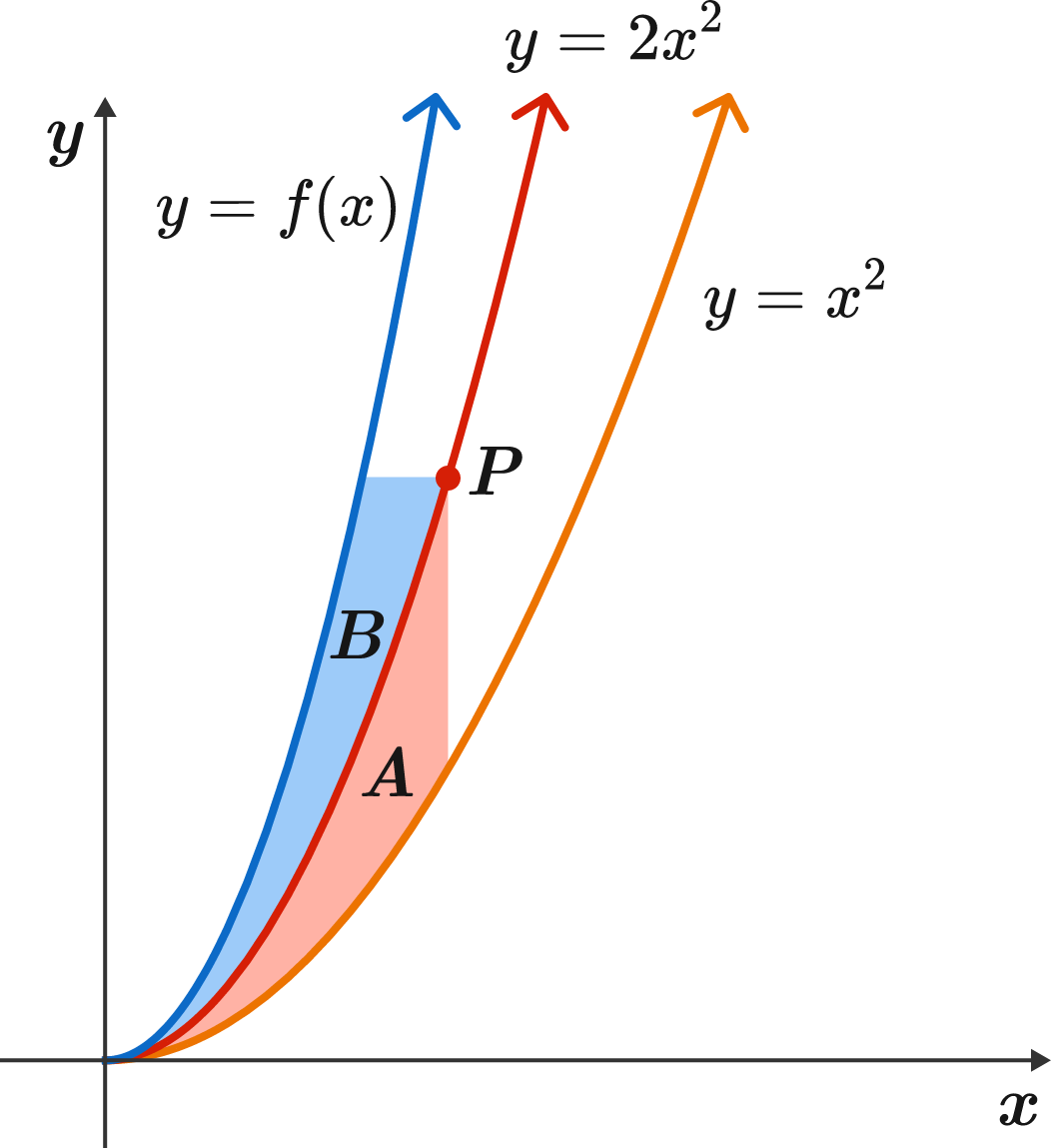# Matching Areas

Calculus Level 3

Take a point $P$ on the graph of $y=2x^2,$ and draw a line going through $P$ parallel to the $y$-axis. Then call the red area $A:$ the area bounded by this line, the graph of $y=x^2,$ and the graph of $y=2x^2.$

Now, draw a line going through $P$ parallel to the $x$-axis. Then call the blue area $B:$ the area bounded by this line, the graph of $y=2x^2,$ and the graph of $y=f(x).$

This function $f(x)$ is continuous and displays the unique property that for every point $P$ on $y = 2x^2,$ the two areas $A$ and $B$ are equal.

Find $f(x)$ and evaluate $f(12).$×

Problem Loading...

Note Loading...

Set Loading...